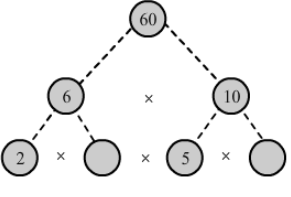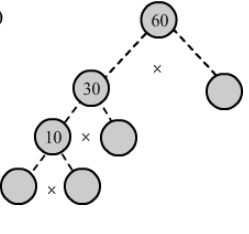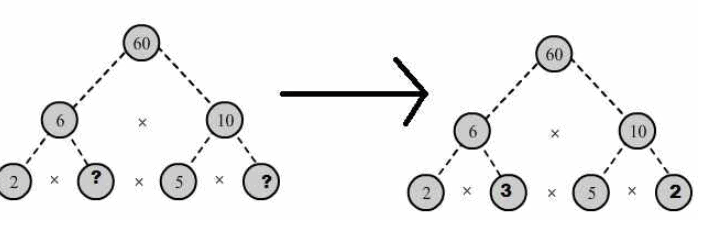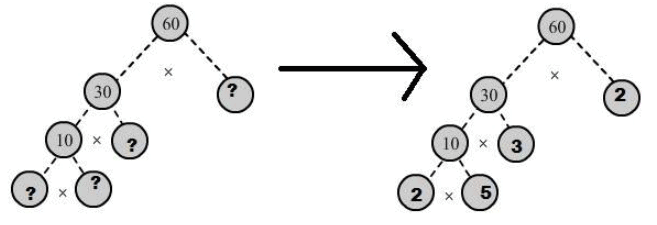Courses

# RD Sharma Solutions: Exercise 2.4- Playing With Numbers Class 6 Notes | EduRev

## Class 6 : RD Sharma Solutions: Exercise 2.4- Playing With Numbers Class 6 Notes | EduRev

The document RD Sharma Solutions: Exercise 2.4- Playing With Numbers Class 6 Notes | EduRev is a part of the Class 6 Course Mathematics (Maths) Class 6.
All you need of Class 6 at this link: Class 6

Q.1. In which of the following expressions, prime factorization has been done?

(i) 24 = 2 × 3 × 4

(ii) 56 = 1 × 7 × 2 × 2 × 2

(iii) 70 = 2 × 5 × 7

(iv) 54 = 2 × 3 × 9

Ans:

(i) 24 = 2 × 3 × 4 is not a prime factorisation as 4 is not a prime number.

(ii) 56 = 1 × 7 × 2 × 2 × 2 is not a prime factorisation as 1 is not a prime number.

(iii) 70 = 2 × 5 × 7 is a prime factorisation as 2, 5, and 7 are prime numbers.

(iv) 54 = 2 × 3 × 9 is not a prime factorisation as 9 is not a prime number.

Q.2. Determine prime factorization of each of the following numbers:

(i) 216

(ii) 420

(iii) 468

(iv) 945

(v) 7325

(vi) 13915

Ans:

(i) 216

We have:∴ Prime factorisation of 216 = 2 × 2 × 2 × 3 × 3 × 3

(ii) 420

We have:∴ Prime factorisation of 420 = 2 × 2 × 3 × 5 × 7

(iii) 468

We have:∴ Prime factorisation of 468 = 2 × 2 × 3 × 3 × 13

(iv) 945

We have:∴ Prime factorisation of 945 = 3 × 3 × 3 × 5 × 7

(v) 7325

We have:∴ Prime factorisation of 7325 = 5 × 5 × 293

(vi) 13915

We have:∴ Prime factorisation of 13915 = 5 × 11 × 11 × 23

Q.3. Write the smallest 4-digit number and express it as a product of primes.

Ans: The smallest 4-digit number is 1000.

1000 = 2 × 500

= 2 × 2 × 250

= 2 × 2 × 2 × 125

= 2 × 2 × 2 × 5 × 25

= 2 × 2 × 2 × 5 × 5 × 5

∴ 1000 = 2 ×2 × 2 × 5 × 5 × 5

Q.4. Write the largest 4-digit number and give its prime factorization.

Ans: The largest 4-digit number is 9999.

We have:Hence, the largest 4-digit number 9999 can be expressed in the form of its prime factors as 3 × 3 × 11 × 101.

Q.5. Find the prime factors of 1729. Arrange the factors in ascending order, and find the relation between two consecutive prime factors.

Ans: The given number is 1729.

We have:Thus, the number 1729 can be expressed in the form of its prime factors as 7 ×13 ×19.

Relation between its two consecutive prime factors:

The consecutive prime factors of the given number are 7, 13, and 19.

Clearly, 13 − 7 = 6 and 19 − 13 = 6

Here, in two consecutive prime factors, the latter is 6 more than the previous one.

Q.6. Which factors are not included in the prime factorization of a composite number?

Ans: 1 and the number itself are not included in the prime factorisation of a composite number.

Example: 4 is a composite number.

Prime factorisation of 4 = 2 × 2

Q.7. Here are two different factor trees for 60. Write the missing numbers:

(i)(ii)Ans: (i) Since 6 = 2 × 3 and 10 = 5 × 2, we have:(ii) Since 60 = 30 × 2, 30 = 10 × 3 and 10 = 5 × 2, we have:Offer running on EduRev: Apply code STAYHOME200 to get INR 200 off on our premium plan EduRev Infinity!

## Mathematics (Maths) Class 6

191 videos|224 docs|43 tests

,

,

,

,

,

,

,

,

,

,

,

,

,

,

,

,

,

,

,

,

,

;